• 简单线性回归 文章目录简单线性回归一、最小二乘法二、实现简单的线性回归算法三、使用面向对象编程实现四、向量化运算五、线性回归算法的评价1、均方误差(MSE)2、均方根误差(RMSE)3、平均绝对误差(MAE)六、最好的...
简单线性回归

文章目录简单线性回归一、最小二乘法二、实现简单的线性回归算法三、使用面向对象编程实现四、向量化运算五、线性回归算法的评价1、均方误差(MSE)2、均方根误差(RMSE)3、平均绝对误差(MAE)六、最好的衡量线性回归算法的指标我是尾巴

思想简单，是许多强大的非线性模型的基础，具有很好的可解释性。背后有强大的数学理论支持。
其本质：寻找一条直线，最大程度地去拟合样本特征和样本输出标记之间的关系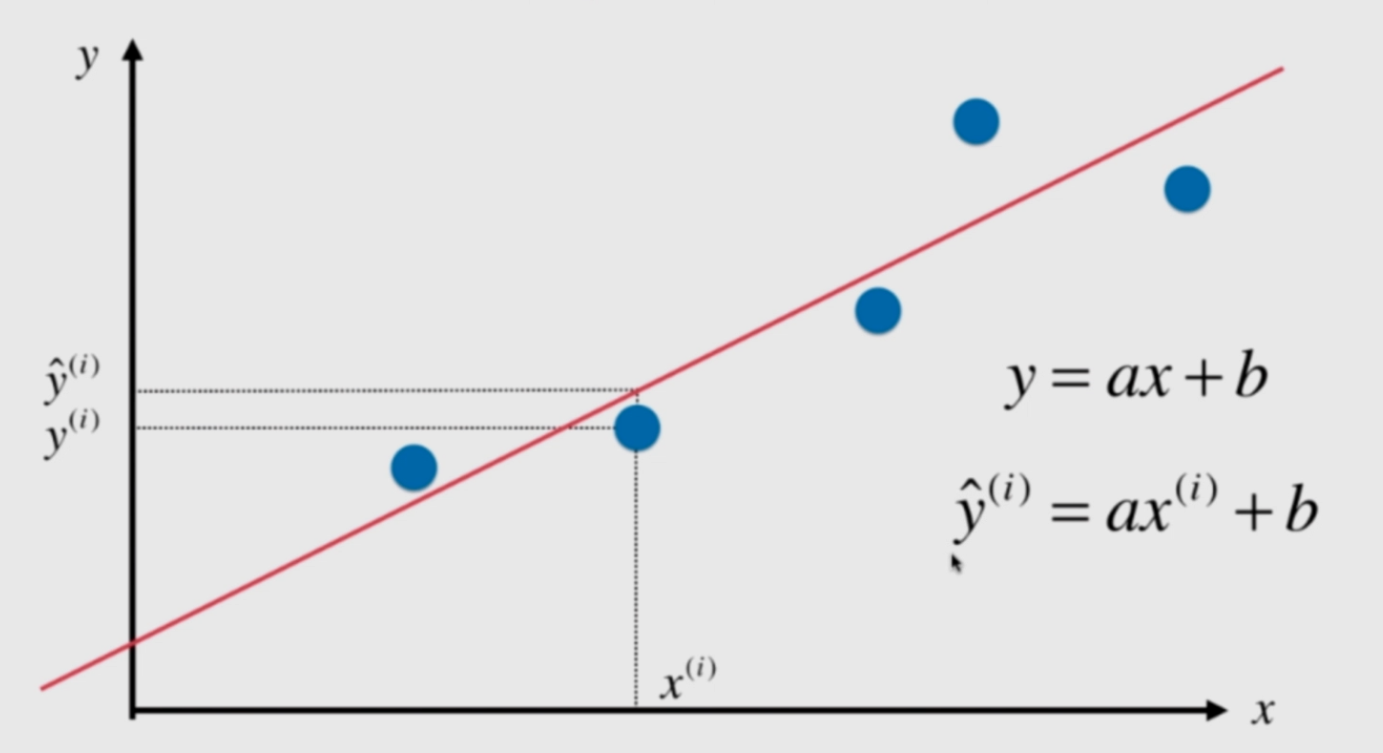​		之所以称为简单线性回归是因为样本特征只有一个。假设我们已经找到最佳拟合直线方程y=ax+b，对于每个样本点x(i)>根据直线方程我们就能预测出y(i),其真值为y，我们希望预测值与真值尽可能的小，y(i)>-y尽可能的小，但是其有可能有有负，因此我们将其进行平方。并且这个函数处处可导。
​		目标：使$\sum_{i=1}^m$(y-y(i))2尽可能的小，
​		目标：找到a和b使得$\sum_{i=1}^m$(y-ax+b)2尽可能小
​		在机器学习中我们把上述的目标函数称为损失函数(loss function)或者称为效用函数(utility function),通过分析问题，确定损失函数或者效用函数，通过优化这些目标函数，最终获得机器学习模型。
​		其实上述的目标函数就是一个最典型的最小二乘法问题，最下化误差的平方。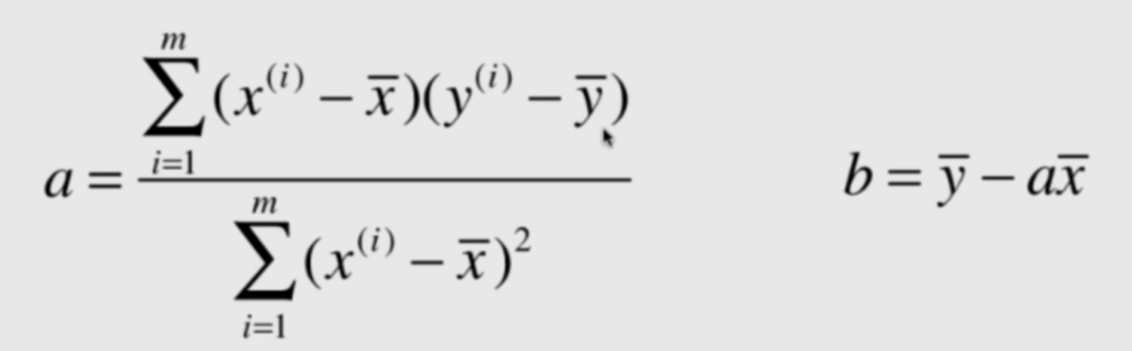一、最小二乘法
目标：找到a和b使得$\sum_{i=1}^m$(y-ax+b)2尽可能小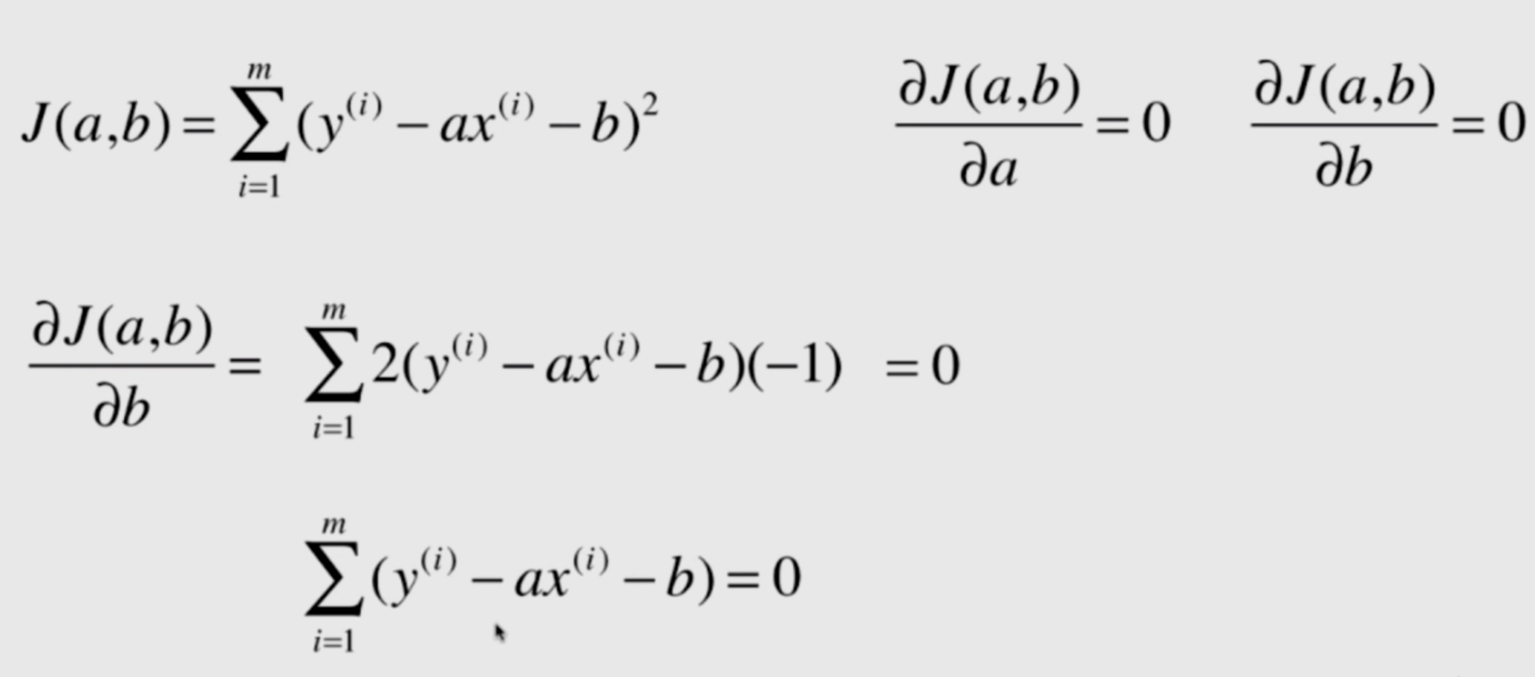分别对a和b求偏导，并令其等于0。首先对b进行求偏导，b的计算较为简单。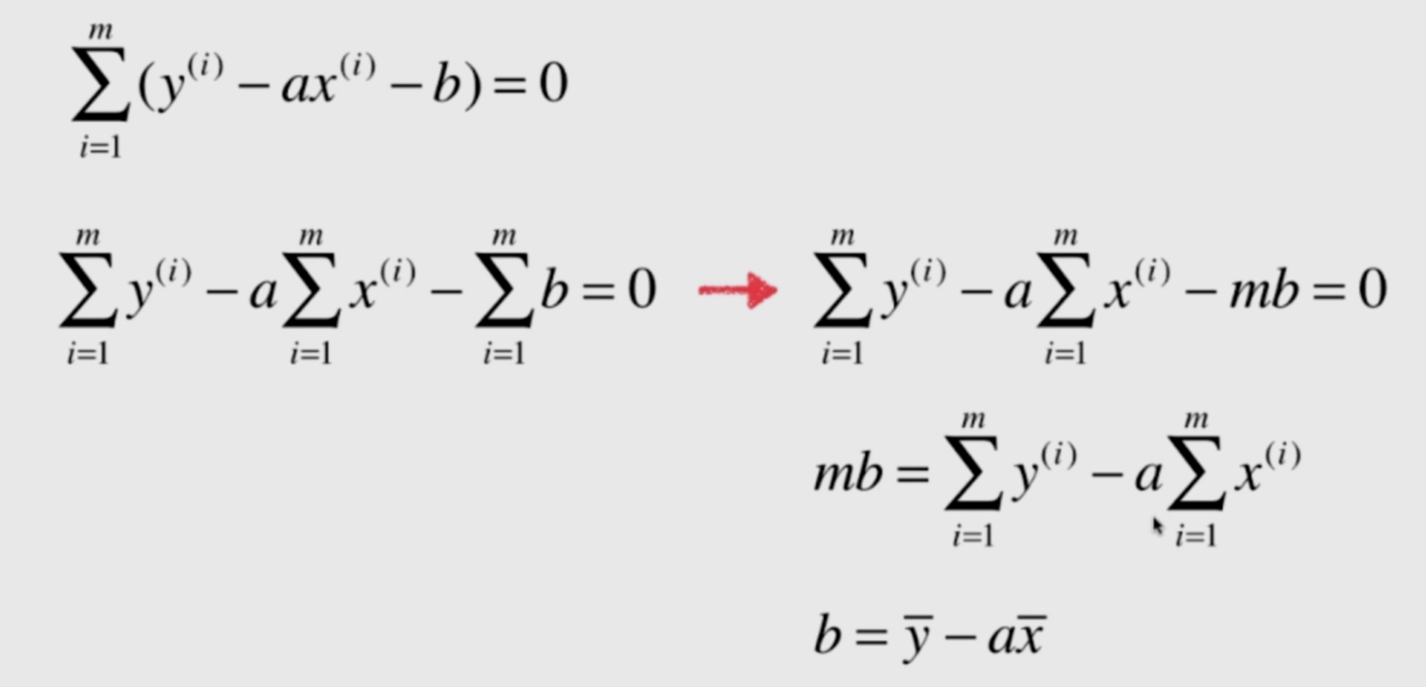接下来对a求偏导。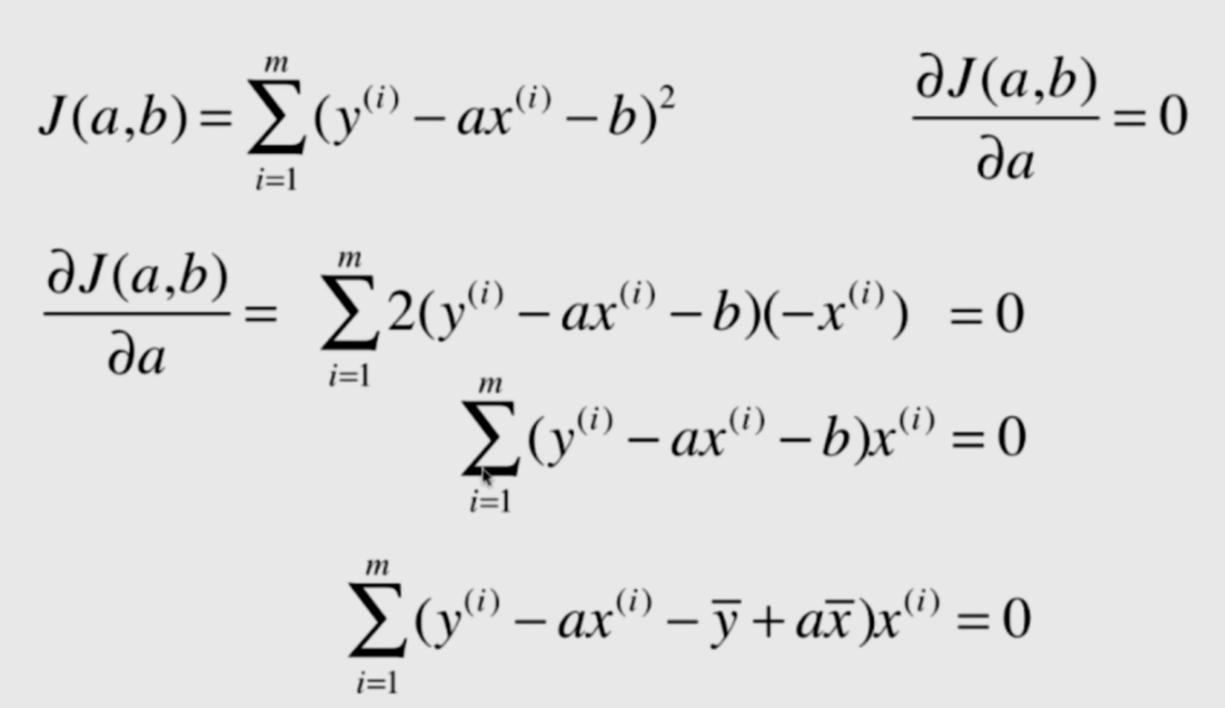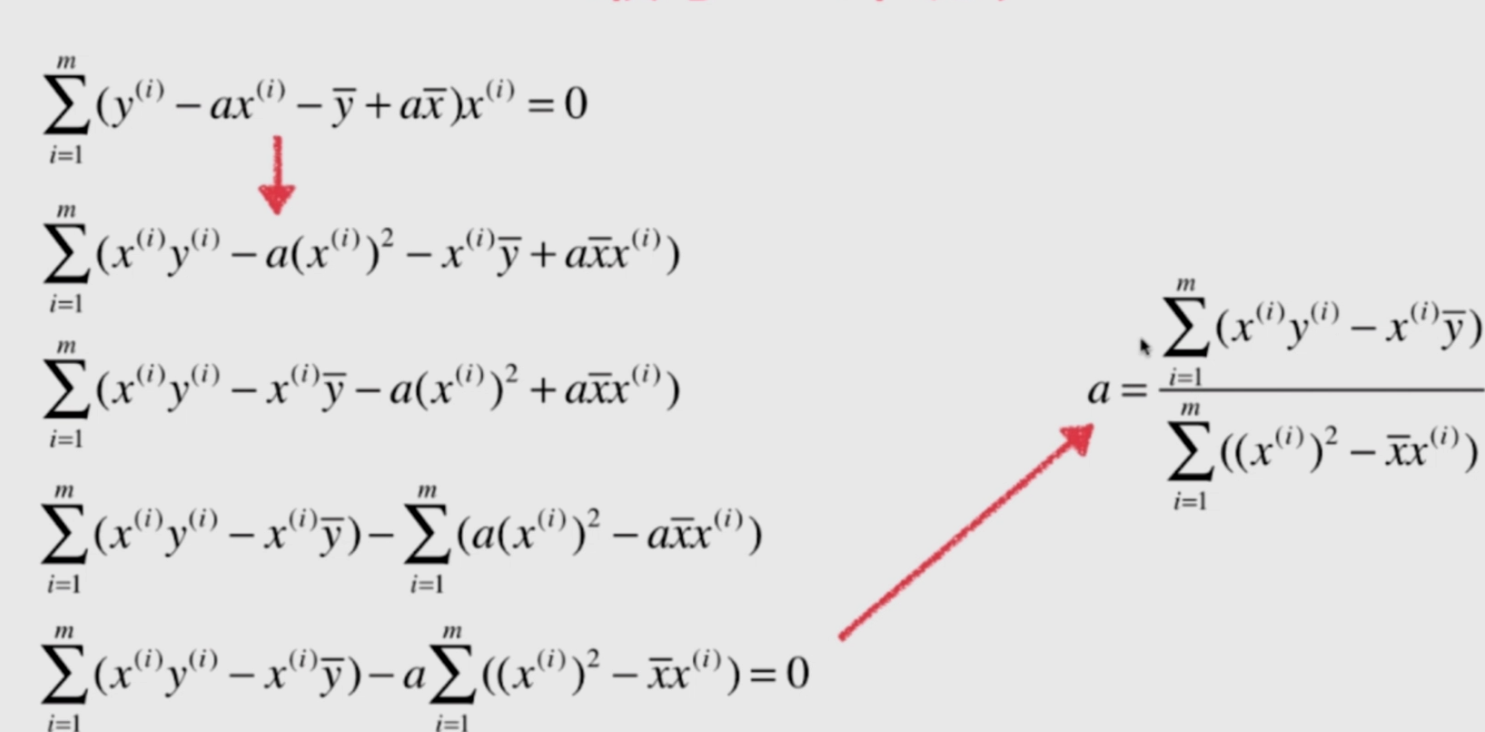​		其实到这里，已经推到完了，a和b都有了自己的公式，就可以编程实现了。但是，往往在学习过程中，我们还会在把它化简一步。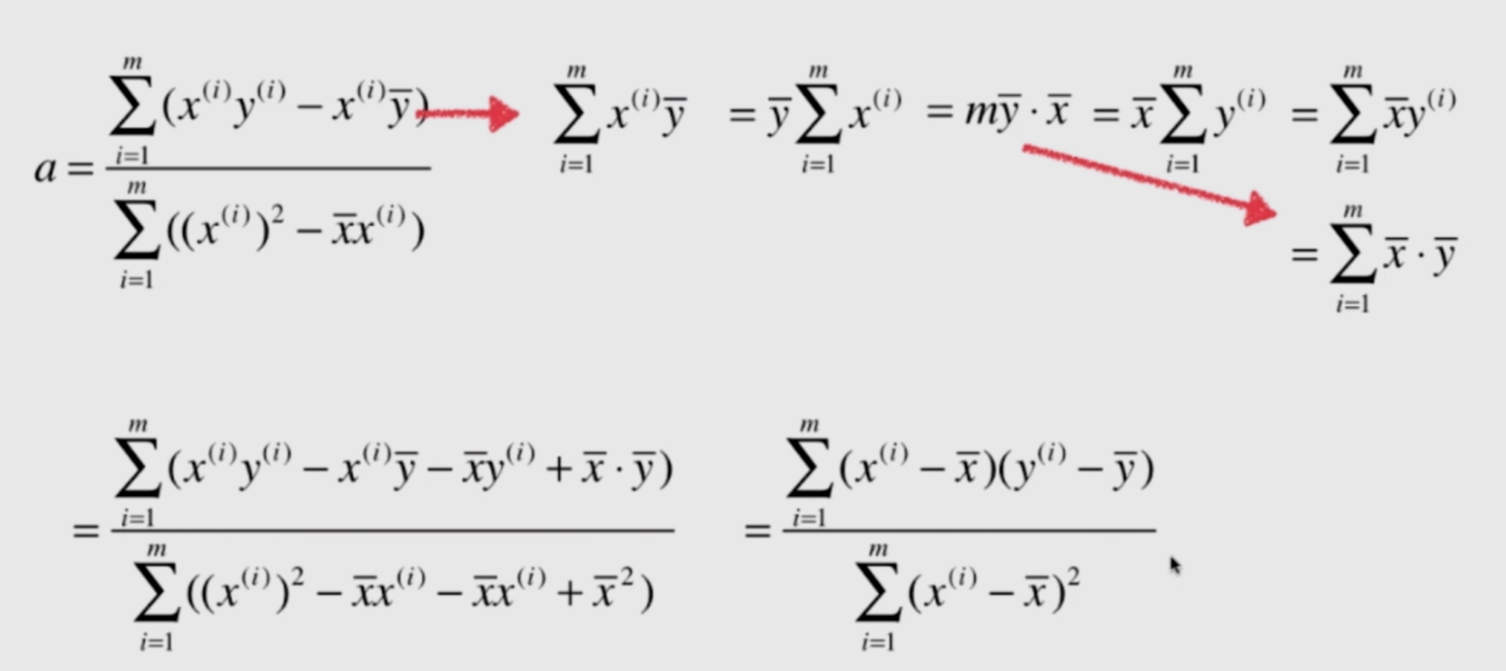​		这样的到a其实是为了更加方便使用向量化运算能够缩短程序的运行时间，效率高20倍以上。
二、实现简单的线性回归算法
​		准备一些简单的数据。
import numpy as np
import matplotlib.pyplot as plt

x = np.array([1., 2., 3., 4., 5.])
y = np.array([1., 3., 2., 3., 5.])

plt.scatter(x, y)
plt.axis([0, 6, 0, 6])
plt.show()

x_mean = np.mean(x)
y_mean = np.mean(y)
up = 0
down = 0
for x_i, y_i in zip(x, y):
up += (x_i - x_mean) * (y_i - y_mean)
down += (x_i - x_mean) ** 2
a = up/down
b = y_mean - a * x_mean

​		这样我们就求出了a和b，也就求出了直线方程y=a+b
y_hat = a * x + b
plt.scatter(x, y)
plt.plot(x, y_hat, 'r')
plt.axis([0, 6, 0, 6])
plt.show()
# 接下来测试一个新的数据
x_new = 6
y_predict = a * x_new + b

输出结果：5.2
三、使用面向对象编程实现
import numpy as np

class SimpleLinearRegreesion1(object):

def __init__(self):
self.a_ = None
self.b_ = None

def fit(self, x_train, y_train):
assert x_train.ndim == 1, "Simple linear regression can only solve single feature training data"
assert len(x_train) == len(y_train), "the size of x_train must be equal to the size of y_train"

x_mean = np.mean(x_train)
y_mean = np.mean(y_train)

up = 0
down = 0
for x_i, y_i in zip(x_train, y_train):
up += (x_i - x_mean) * (y_i - y_mean)
down += (x_i - x_mean) ** 2

self.a_ = up / down
self.b_ = y_mean - self.a_ * x_mean
return self

def predict(self, x_predict):
assert x_predict.ndim == 1, "Simple linear regression can only solve single feature training data"
assert self.a_ is not None and self.b_ is not None, "must fit before predict!"
"这个函数用来处理一次传入多个x_predict"
return [self._predict(x) for x in x_predict]

def _predict(self, x_single):
"预测单个数据"
return self.a_ * x_single + self.b_

def __repr__(self):
return "SimpleLinearRegression"

if __name__ == '__main__':
import numpy as np

x = np.array([1., 2., 3., 4., 5.])
y = np.array([1., 3., 2., 3., 5.])
x_new = 6

reg1 = SimpleLinearRegreesion1()
reg1.fit(x, y)
y_predict = reg1.predict(np.array([x_new]))
print(y_predict)
print(reg1.a_)
print(reg1.b_)

四、向量化运算
import numpy as np

class SimpleLinearRegreesion2(object):

def __init__(self):
self.a_ = None
self.b_ = None

def fit(self, x_train, y_train):
assert x_train.ndim == 1, "Simple linear regression can only solve single feature training data"
assert len(x_train) == len(y_train), "the size of x_train must be equal to the size of y_train"

x_mean = np.mean(x_train)
y_mean = np.mean(y_train)

up = (x_train - x_mean).dot(y_train - y_mean)
down = (x_train - x_mean).dot((x_train - x_mean))

self.a_ = up / down
self.b_ = y_mean - self.a_ * x_mean
return self

def predict(self, x_predict):
assert x_predict.ndim == 1, "Simple linear regression can only solve single feature training data"
assert self.a_ is not None and self.b_ is not None, "must fit before predict!"
"这个函数用来处理一次传入多个x_predict"
return [self._predict(x) for x in x_predict]

def _predict(self, x_single):
"预测单个数据"
return self.a_ * x_single + self.b_

def __repr__(self):
return "SimpleLinearRegression"

​		接下来进行性能测试：
if __name__ == '__main__':
import numpy as np
import time

m = 100000
x = np.random.random(size=m)
y = x * 2.0 + 3.0 + np.random.normal(0, 1, size=m)

start1 = time.time()
reg1 = SimpleLinearRegreesion1()
reg1.fit(x, y)
stop1 = time.time()
print("运行时间:%s" % (stop1 - start1))

start2 = time.time()
reg2 = SimpleLinearRegreesion2()
reg2.fit(x, y)
stop2 = time.time()
print("运行时间:%s" % (stop2 - start2))

输出结果：
运行时间:0.07222509384155273
运行时间:0.008754968643188477
五、线性回归算法的评价
1、均方误差(MSE)
​		均方误差（Mean Squared Error）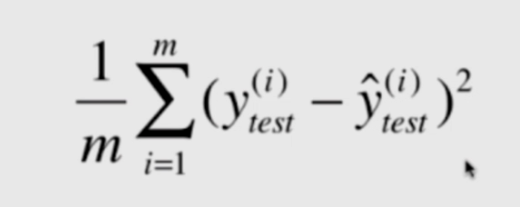2、均方根误差(RMSE)
​		均方根误差（Root Mean Squared Error）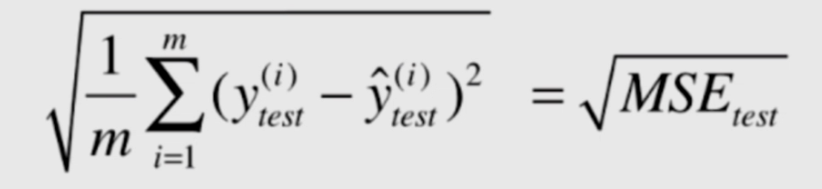​		这样，MSE就和y有着相同的量纲。
3、平均绝对误差(MAE)
​		平均绝对误差（Mean Absolute Error）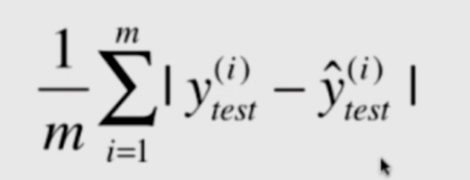我们在最初的时候选择目标函数不是它，因为绝对值不是一个处处可导的函数，因此不能作为损失函数，但是作为评价函数还是相当不错的。
​		接下里使用波士顿房价做简单线性回归，并使用这面三中评价函数对线性回归模型进行评价。
import numpy as np
import matplotlib.pyplot as plt
from sklearn import datasets

boston = datasets.load_boston()
print(boston.DESCR)
boston.feature_names
x = boston.data[:, 5]   # 只是用房间数量这个特征
y = boston.target		# 波士顿房价
plt.scatter(x, y)
plt.show()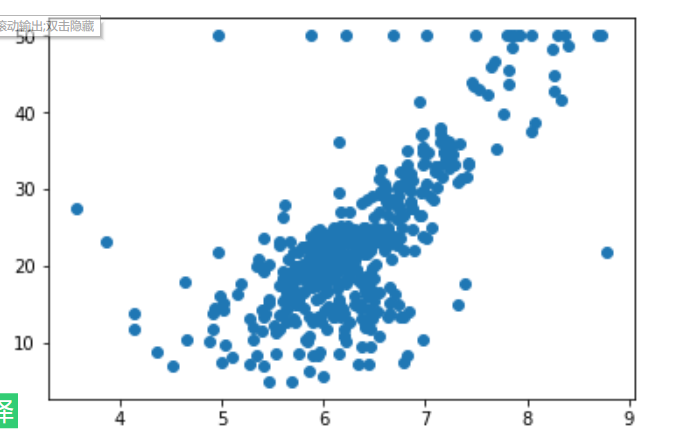​		通过可视化发现最上方有一排异常点，所以在数据处理的过程中我们需要考虑，可能是因为在统计的过程中设计了上限，就是大于50万的都按50万计算，因此，数据的预处理就显得尤为关键。
x = x[y < np.max(y)]
y = y[y < np.max(y)]
plt.scatter(x, y)
plt.show()

from sklearn.model_selection import train_test_split

x_train, x_test, y_train, y_test = train_test_split(x, y, random_state=666)
x_train.shape
x_test.shape
%run D:/goodgoodstudy/linear_regression/simple_linear_regression.py
reg = SimpleLinearRegreesion2()
reg.fit(x_train, y_train)
reg.a_
reg.b_
plt.scatter(x_train, y_train)
plt.plot(x_train, reg.predict(x_train), 'r')
plt.show()


​		接下来测试三种评价函数：
y_predict = reg.predict(x_test)
mes_test = np.sum((y_predict - y_test)**2) / len(y_test)
mes_test

​		输出结果：28.215949368640796
from math import sqrt
rmese_test = sqrt(mes_test)
rmese_test

​		输出结果：5.311868726600912
mae_test = np.sum(np.absolute(y_predict - y_test)) / len(y_test)
mae_test


​		输出结果：3.9489046062737843
使用函数把这三个评价函数封装一下：
import numpy as np
from math import sqrt

def mean_square_error(y_true, y_predict):
assert len(y_true) == len(y_predict), "the size of y_true must be equal to the size of y_predict"

return np.sum((y_predict - y_true)**2) / len(y_true)

def root_mean_square_error(y_true, y_predict):
return sqrt(mean_square_error(y_true, y_predict))

def mean_absolute_error(y_true, y_predict):
assert len(y_true) == len(y_predict), "the size of y_true must be equal to the size of y_predict"

return np.sum(np.absolute(y_predict - y_true)) / len(y_true)

​		其实，在sklearn中又已经封装好了，那威慑呢么还要写一遍呢？因为不能仅仅只会调库，底层代码还是要知道是怎么实现的。那么我们看看sklearn中封装的函数和自己写的输出是否一致。
from sklearn.metrics import mean_absolute_error
from sklearn.metrics import mean_squared_error

mean_absolute_error(y_test, y_predict)
mean_squared_error(y_test, y_predict)

六、最好的衡量线性回归算法的指标
R squared
​			前面已经讲了三个评价线性回归模型的函数，为什么还会有最好的衡量线性回归算法的指标呢？我们想一下分类问题。1是完全正确，0是错误，这样我们就能大概知道分类的情况，但是如果使用上面三个评价函数，我们并不能知道差了多少。只知道有误差，所以我们就像办法把误差范围也归一到0-1之间。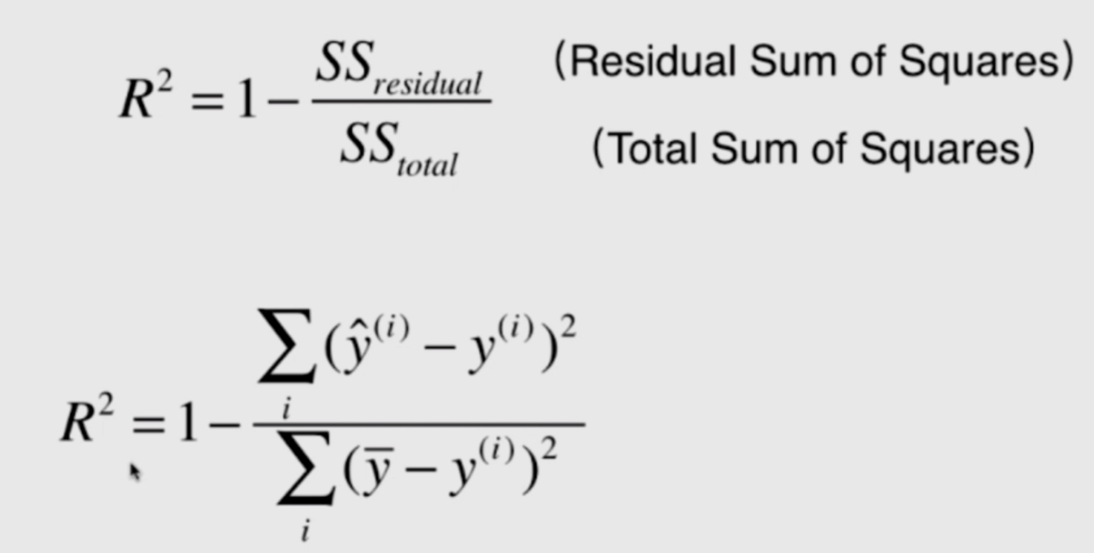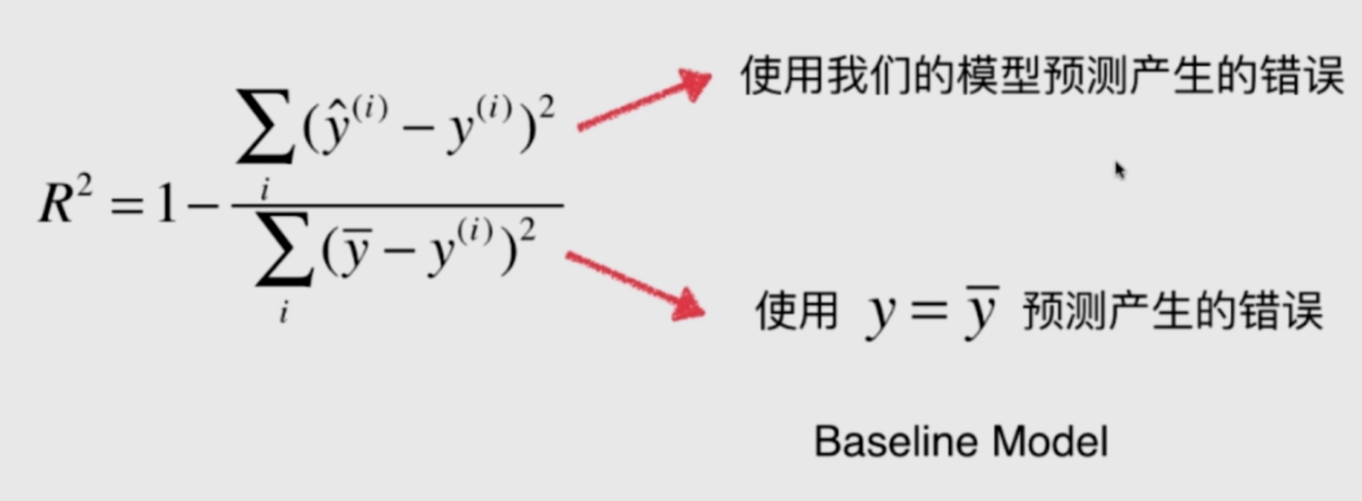​		如果我们的模型预测产生的错误为0或者很小也就是后面一项基本为0，就是R约等于1，模型很好。如果模型预测产生的错误很大，基本跟用均值预测产生的误差接近，后面一项基本为1，就是R越等与0，模型很差。如果我们的模型预测产生的错误比用均值产生的错误还大，那就没什么意义了，还不如直接用均值预测，那就没必要了，**如果R小于0，很有可能就是因为我们的数据根本不存在线性关系**。因此R一定不会小于0，因此，我们成用均值预测的模型称为Baseline Model。这就是底线，基本不用学习就可以通过统计得到的模型。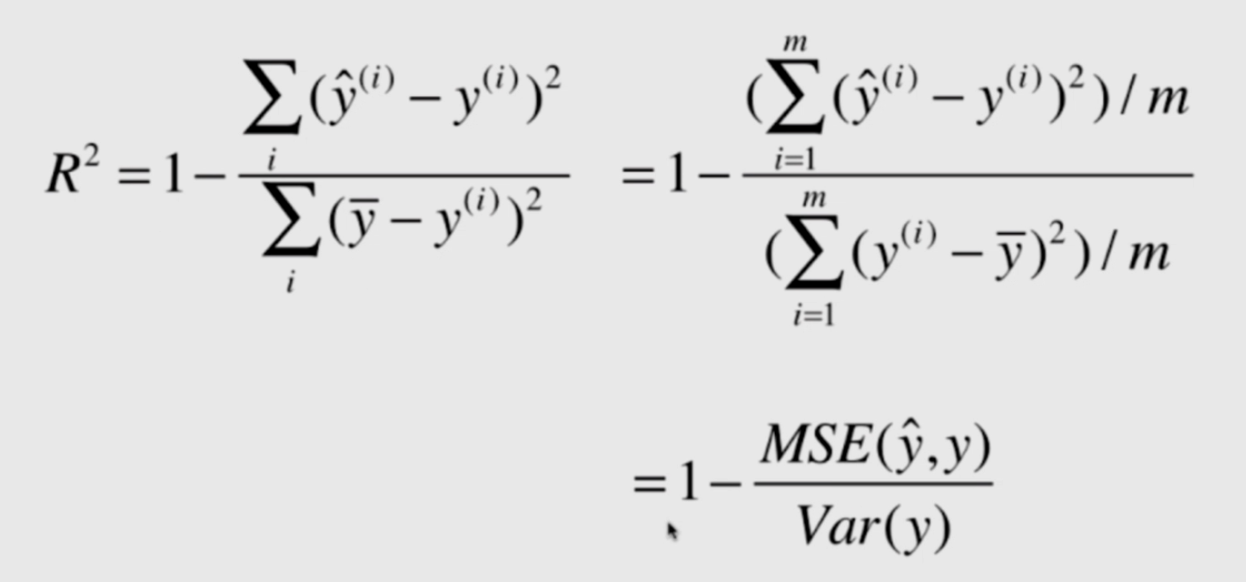​		通过上面这个式子，可以发现R[^2]背后还存在着重大的数学统计意义。接下来就用编程去实现它。
def r2_score(y_true, y_predict):

return 1 - mean_square_error(y_true, y_predict) / np.var(y_true)

sklearn中：
from sklearn.metrics import r2_score

r2_score(y_test, y_predict)

最后，我们再把这个加到我们封装好的简单线性回归的类中。
import numpy as np
from .metrics import r2_score

class SimpleLinearRegreesion1(object):

def __init__(self):
self.a_ = None
self.b_ = None

def fit(self, x_train, y_train):
assert x_train.ndim == 1, "Simple linear regression can only solve single feature training data"
assert len(x_train) == len(y_train), "the size of x_train must be equal to the size of y_train"

x_mean = np.mean(x_train)
y_mean = np.mean(y_train)

up = 0
down = 0
for x_i, y_i in zip(x_train, y_train):
up += (x_i - x_mean) * (y_i - y_mean)
down += (x_i - x_mean) ** 2

self.a_ = up / down
self.b_ = y_mean - self.a_ * x_mean
return self

def predict(self, x_predict):
assert x_predict.ndim == 1, "Simple linear regression can only solve single feature training data"
assert self.a_ is not None and self.b_ is not None, "must fit before predict!"
"这个函数用来处理一次传入多个x_predict"
return [self._predict(x) for x in x_predict]

def _predict(self, x_single):
"预测单个数据"
return self.a_ * x_single + self.b_

def __repr__(self):
return "SimpleLinearRegression"

class SimpleLinearRegreesion2(object):

def __init__(self):
self.a_ = None
self.b_ = None

def fit(self, x_train, y_train):
assert x_train.ndim == 1, "Simple linear regression can only solve single feature training data"
assert len(x_train) == len(y_train), "the size of x_train must be equal to the size of y_train"

x_mean = np.mean(x_train)
y_mean = np.mean(y_train)

up = (x_train - x_mean).dot(y_train - y_mean)
down = (x_train - x_mean).dot((x_train - x_mean))

self.a_ = up / down
self.b_ = y_mean - self.a_ * x_mean
return self

def predict(self, x_predict):
assert x_predict.ndim == 1, "Simple linear regression can only solve single feature training data"
assert self.a_ is not None and self.b_ is not None, "must fit before predict!"
"这个函数用来处理一次传入多个x_predict"
return [self._predict(x) for x in x_predict]

def _predict(self, x_single):
"预测单个数据"
return self.a_ * x_single + self.b_

def score(self, x_test, y_test):

y_predict = self.predict(x_test)
return r2_score(y_test, y_predict)

def __repr__(self):
return "SimpleLinearRegression"

​		最后，简单线性回归就到这里了！想法思想很简单，但是其中数学统计知识还是值得我们学习和思考的。
我是尾巴本次推荐：集万千工具为一体的网站。
http://tool.uixsj.cn/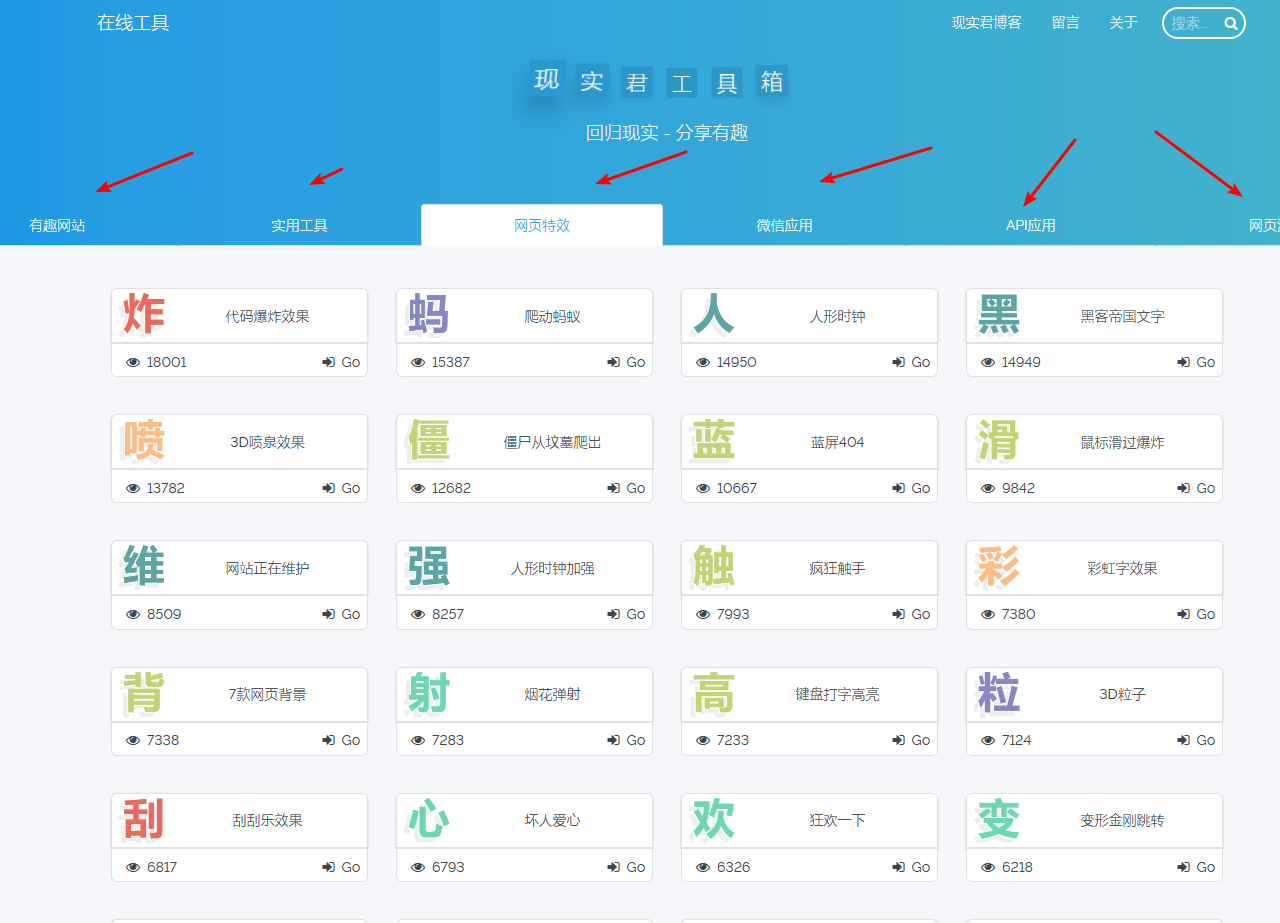继续加油！！！今天又是元气满满的一天！


展开全文• 使用Python进行简单线性回归 在ML算法中，我们有： 监督学习 无监督学习 在监督学习中，我们有： 回归 分类 首先，我们讨论不同类型的回归。 线性回归 简单线性回归 多元线性回归 逻辑回归 套索回归 岭回归 在此...
• 当只有一个自变量时，称为简单线性回归，当具有多个自变量时，称为多元线性回归。 线性关系的理解： 画出来的图像是直的。 每个自变量的最高次项为1。 拟合是指构建一种算法，使得该算法能够符合真实的数据。从...
回归分析是用来评估变量之间关系的统计过程。用来解释自变量X与因变量Y的关系。即当自变量X发生改变时，因变量Y会如何发生改变。
线性回归是回归分析的一种，评估的自变量X与因变量Y之间是一种线性关系。当只有一个自变量时，称为简单线性回归，当具有多个自变量时，称为多元线性回归。
线性关系的理解：

画出来的图像是直的。
每个自变量的最高次项为1。

拟合是指构建一种算法，使得该算法能够符合真实的数据。从机器学习角度讲，线性回归就是要构建一个线性函数，使得该函数与目标值之间的相符性最好。从空间的角度来看，就是要让函数的直线（面），尽可能靠近空间中所有的数据点（点到直线的平行于y轴的距离之和最短）。线性回归会输出一个连续值。
线性回归模型
简单线性回归
我们可以以房屋面积（x）与房价（y）为例，二者是线性关系，房屋价格正比于房屋面积，假设比例为w：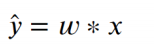然而，这种线性方程一定是过原点的，即x为0时，y也一定为0。这可能并不符合现实中某些场景。为了能够让方程具有更广泛的适应性，就要再增加一个截距，设为b，则方程可以变为：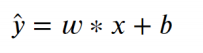以上方程就是数据建模的模型，w与b就是模型的参数。
线性回归是用来解释自变量与因变量之间的关系，但这种关系并非严格的函数映射关系。
多元线性回归
现实中的数据可能是比较复杂的，自变量也可能不止一个，例如，影响房屋价格也很可能不止房屋面积一个因素，可能还有是否在地铁附近，房间数，层数，建筑年代等诸多因素。不过，这些因素对房价影响的权重是不同的，因此，我们可以使用多个权重来表示多个因素与房屋价格的关系：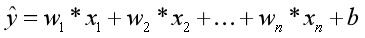x：影响因素，即特征。
w：每个x的影响力度。
n：特征的个数。
y^：房屋的预测价格。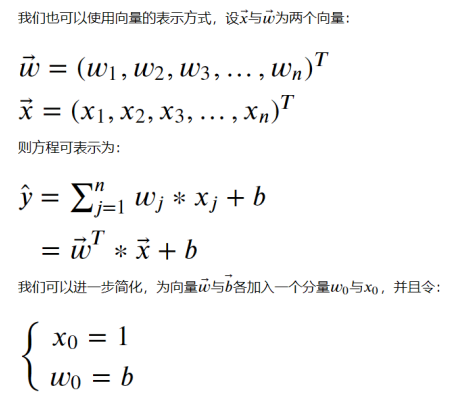这样，就可以表示为：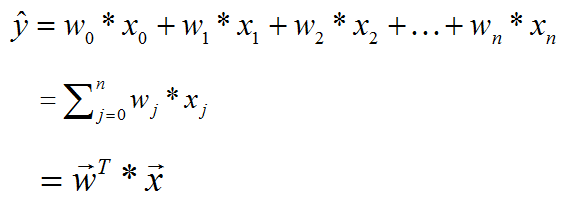多元线性回归在空间中，可以表示为一个超平面，去拟合空间中的数据点。
我们的目的就是从现有的数据中，去学习w与b的值。一旦w与b的值确定，就能够确定拟合数据的线性方程，这样就可以对未知的数据x进行预测y（房价）。
损失函数与参数求解
损失函数
对于机器学习来讲，就是从已知数据去建立一个模型，使得该模型能够对未知数据进行预测。实际上，机器学习的过程就是确定模型参数的过程。
对于监督学习来说，我们可以通过建立损失函数来实现模型参数的求解，损失函数也称目标函数或代价函数，简单来说就是关于误差的一个函数。损失函数用来衡量模型预测值与真实值之间的差异。机器学习的目标，就是要建立一个损失函数，使得该函数的值最小。
也就是说，损失函数是一个关于模型参数的函数（以模型参数w作为自变量的函数），自变量可能的取值组合通常是无限的，我们的目标，就是要在众多可能的组合中，找到一组最合适的自变量组合，使得损失函数的值最小。
在线性回归中，我们使用平方损失函数（最小二乘法），定义如下：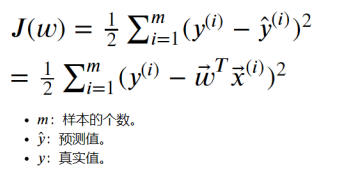简单线性回归程序
我们以鸢尾花数据集中花瓣长度与花瓣宽度为例，通过程序实现简单线性回归。
import numpy as np
#用于线性回归的类
from sklearn.linear_model import LinearRegression
#用来切分训练集与测试集
from sklearn.model_selection import train_test_split
from sklearn.datasets import load_iris
#设置输出精度。默认为8
np.set_printoptions(precision=2)

iris = load_iris()
#获取花瓣长度作为x，花瓣宽度作为y。
x, y = iris.data[:,2].reshape(-1,1), iris.data[:,3]

lr = LinearRegression()
x_train, x_test, y_train, y_test = train_test_split(x, y, test_size=0.25, random_state=0)
#使用训练集数据，训练模型。
lr.fit(x_train, y_train)
print('权重：', lr.coef_)
print('截距：', lr.intercept_)
y_hat = lr.predict(x_test)
print('实际值：', y_test[:5])
print('预测值：', y_test[:5])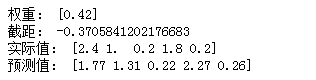import matplotlib.pyplot as plt

plt.rcParams['font.family'] = 'SimHei'
plt.rcParams['axes.unicode_minus'] = False
plt.rcParams['font.size'] = 15

plt.figure(figsize=(10,6))
plt.scatter(x_train, y_train, c='orange', label='训练集')
plt.scatter(x_test, y_test, c='g', marker='D', label='测试集')
plt.plot(x, lr.predict(x), 'r-')
plt.legend()
plt.xlabel('花瓣长度')
plt.ylabel('花瓣宽度')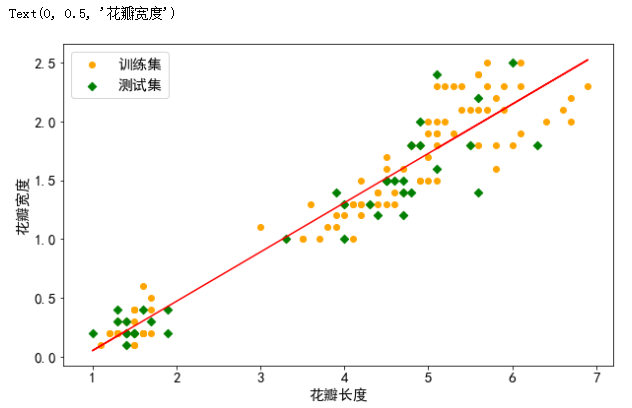plt.figure(figsize=(15,6))
plt.plot(y_test, label='真实值', color='r', marker='o')
plt.plot(y_hat, c='g', marker='o', ls='--', label='预测值')
plt.legend()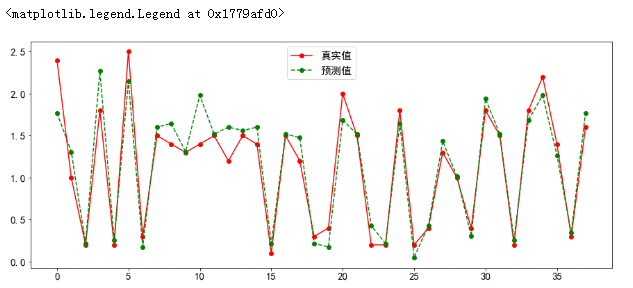回归模型预测
对于回归模型，我们可以采用如下指标进行衡量。

MSE
RMSE
MAE
R2

MSE
MSE（Mean Squared Error），平均平方差，为所有样本数据误差（真实值与预测值之差）的平方和，然后取均值。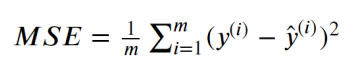RMSE
RMSE（Root Mean Squared Error），平均平方误差的平方根，即在MSE的基础上，取平方根。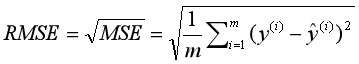MAE
MAE（Mean Absolute Error），平均绝对值误差，为所有样本数据误差的绝对值和。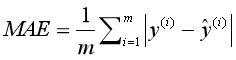R2
R2为决定系数，用来表示模型拟合性的分值，值越高表示模型拟合性越好，在训练集中，R2的取值范围为[0,1]。在R2的取值范围为[-∞,1]。
R2的计算公式为1减去RSS与TSS的商。其中，TSS（Total Sum of Squares）为所有样本数据与均值的差异，是方差的m倍。而RSS（Residual sum of Squares）为所有样本数据误差的平方和，是MSE的m倍。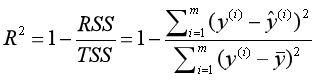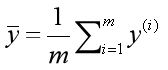从公式定义可知，最理想情况，所有的样本数据的预测值与真实值相同，即RSS为0，此时R2为1。
from sklearn.metrics import mean_squared_error, mean_absolute_error, r2_score

print('均方误差（MSE）：',mean_squared_error(y_test,y_hat))
print('根均方误差（RMSE）：',np.sqrt(mean_squared_error(y_test,y_hat)))
print('平均绝对值误差（MAE）：',mean_absolute_error(y_test,y_hat))
print('训练集R^2：',r2_score(y_train,lr.predict(X_train)))
print('测试集R^2：',r2_score(y_test,y_hat))
#lr.score其实求解的就是R^2的值，但需要注意，r2_score方法与lr.score方法传递参数的内容是不同的。
print('训练集R^2：',lr.score(X_train,y_train))
print('测试集R^2：',lr.score(X_test,y_test))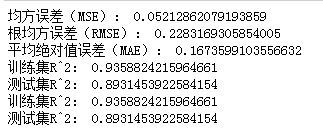多元线性回归程序
我们可以以波士顿房价为例来做程序演示
from sklearn.linear_model import LinearRegression
from sklearn.model_selection import train_test_split
from sklearn.datasets import load_boston
import pandas as pd

boston = load_boston()
x, y = boston.data, boston.target
df = pd.DataFrame(np.concatenate([x, y.reshape(-1,1)], axis=1),
columns = boston.feature_names.tolist()+['MEDV'])
df.head()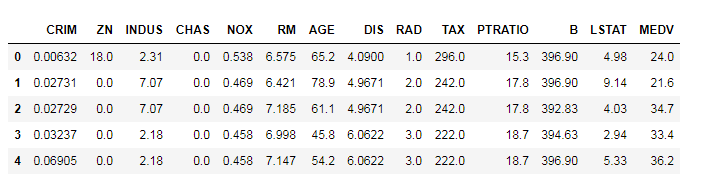x_train, x_test, y_train, y_test = train_test_split(x, y, test_size=0.25, random_state=0)
lr = LinearRegression()
lr.fit(x_train, y_train)
y_hat = lr.predict(x_test)
print('权重：',lr.coef_)
print('截距：',lr.intercept_)
print('实际值：',y_test[:10])
print('测试值：',y_hat[:10])
print('训练值R^2:',lr.score(X_train, y_train))
print('测试值R^2:',lr.score(X_test, y_test))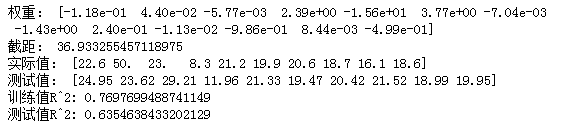展开全文机器学习 python 算法
• 简单线性回归一、什么是线性回归二、线性回归的分类三、一元线性回归的数学知识四、封装一个自己的一元线性回归五、使用自己的一元线性回归预测波士顿房价六、使用sklearn的一元线性回归预测波士顿房价七、回归算法...
简单线性回归一、什么是线性回归二、线性回归的分类三、一元线性回归的数学知识四、封装一个自己的一元线性回归五、使用自己的一元线性回归预测波士顿房价六、使用sklearn的一元线性回归预测波士顿房价七、回归算法的评判方法1、MSE：均方误差2、RMSE均方根误差3、平均绝对误差4、R-squared
一、什么是线性回归
线性回归是指使用一个线性关系来表示数据
我们数学中学习的 y = a*x + b ，就是最典型的线性回归
二、线性回归的分类
根据未知数的数量，线性回归分为：

一元线性回归

多元线性回归

例如：
对于结果 y 而言，若只含有一个未知数 x，就是一元线性回归
若有未知数 x1，x2，x3，x4，就是多元线性回归
三、一元线性回归的数学知识
对于一元线性回归，表达式的行式为：
y = a*x + b
根据数学知识，可以得到：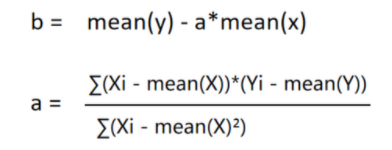mean(x)  表示 X的的平均数

四、封装一个自己的一元线性回归
import numpy as np

class SimpleLinearRegression3:
def __init__(self):
self.a = None
self.b = None

def fit(self, x_arr, y_arr):
assert x_arr.ndim == y_arr.ndim and x_arr.ndim == 1, "the ndim of x_arr and y_arr must be 1"
assert x_arr.shape == y_arr.shape, 'the length of x_arr must be equal to y_arr'
self.x_mean_ = np.mean(x_arr)
self.y_mean_ = np.mean(y_arr)

upper = (x_arr - self.x_mean_).dot(y_arr - self.y_mean_)
down = (x_arr - self.x_mean_).dot(x_arr - self.x_mean_)

self.a = upper / down
self.b = self.y_mean_ - self.a * self.x_mean_

return self

def predict(self, x_preduct):
return np.array([self._predict(x) for x in np.array(x_preduct)])

def _predict(self, x):
return self.a * x + self.b

def score(self,X_test,y_test):
""" R-Squared """
return r2_score(y_test,self.predict(X_test))

def __repr__(self):
return "SimpleLinearRegression3()"


这里的 r2_score() 在下面的评判方法中有具体的介绍和实现代码

五、使用自己的一元线性回归预测波士顿房价
我们使用一元线性回归方法预测一下波士顿的房价
sklearn 中的波士顿房价有多个特征值，这里我们只取出其中的一个特征：房价数量RM
from playML.SimpleLinearRegression import SimpleLinearRegression3
from sklearn import datasets
from sklearn.model_selection import train_test_split
boston = datasets.load_boston()

# 查看数据集的关键字
print(boston.keys())
# dict_keys(['data', 'target', 'feature_names', 'DESCR', 'filename'])

# 查看一下数据集的特征值都有什么
print(boston.feature_names)
# 第六个特征 RM 便是房间数量
# ['CRIM' 'ZN' 'INDUS' 'CHAS' 'NOX' 'RM' 'AGE' 'DIS' 'RAD' 'TAX' 'PTRATIO'
'B' 'LSTAT']

X = boston.data
y = boston.target

X_data = X[y<50][:,5]
y_data = y[y<50]

X_train , X_test , y_train , y_test = train_test_split(X_data , y_data,test_size=0.2,random_state=666)

linear = SimpleLinearRegression3()

linear.fit(X_train , y_train)

y_predict = linear.predict(X_test)

print(y_predict[:10])

print(linear.score(X_test,y_test))
# 0.6129316803937324

六、使用sklearn的一元线性回归预测波士顿房价
这里我们仍然只取出其中的一个特征：房价数量RM
from sklearn.linear_model import LinearRegression
from sklearn import datasets
import numpy as np
from sklearn.model_selection import train_test_split

boston = datasets.load_boston()

X = boston.data
y = boston.target

用散点图的方式简单看一下 RM 一列与房价的大概关系
from matplotlib import pyplot as plt

plt.scatter(X[:,5],y)
plt.show()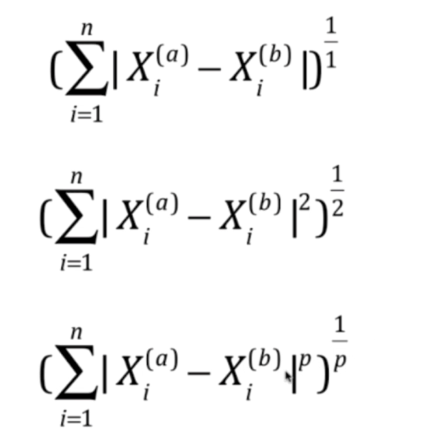可以看到有一小部分数据的房价达到了最大值，这些数据的房价极有可能并不是50，而是50+
所以，我们可以过滤掉这些极端值
# 过滤掉极大值
X_data = X[y<50]
y_data = y[y<50]

# 我们使用的是一元线性回归，所以需要把数据集由矩阵转换为向量
X_data = X_data[:,5].reshape(-1,1)

# 数据集划分为训练集与测试集
X_train , X_test , y_train , y_test = train_test_split(X_data,y_data,test_size=0.2,random_state=666)

# 生成线性回归对象
linear = LinearRegression()

# 训练模型
linear.fit(X_train,y_train)

# 预测数据
y_predict = linear.predict(X_test)
print(y_predict)

# 查看预测的准确率
linear.score(X_test,y_test)
# 0.6129316803937324


因为在数据集中，波士顿房价有13个特征值，而我们在这里进行回归时只取出了一个特征，所以模型预测出来的准确度比较低

七、回归算法的评判方法
1、MSE：均方误差
公式：y(i) 表示实际值
y(j) 表示预测值

自己实现
def mean_squared_error(y_test, y_predict):
"""均方误差 MSE"""
assert len(y_test) == len(y_predict), "the length of y_test must be equal to y_predict"
assert y_test.ndim == 1 and y_predict.ndim == 1, "the ndim of y_test and y_predict must be 1"

return np.sum((y_test - y_predict) ** 2) / len(y_test)


该方法对应于 sklearn 库中的 mean_squared_error( y_test , y_predict )
from sklearn.metrics import mean_squared_error

2、RMSE均方根误差
公式：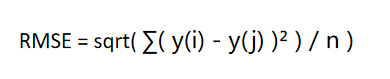def mean_absolute_error(y_test, y_predict):
"""平均绝对误差"""
assert len(y_test) == len(y_predict), "the length of y_test must be equal to y_predict"
assert y_test.ndim == 1 and y_predict.ndim == 1, "the ndim of y_test and y_predict must be 1"

return np.sum(np.abs(y_test - y_predict)) / len(y_test)


sklearn 中没有该评测方法
这种评测方法其实就是 MSE 评分的开方

3、平均绝对误差
公式：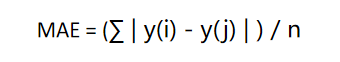def mean_absolute_error(y_test, y_predict):
"""平均绝对误差"""
assert len(y_test) == len(y_predict), "the length of y_test must be equal to y_predict"
assert y_test.ndim == 1 and y_predict.ndim == 1, "the ndim of y_test and y_predict must be 1"

return np.sum(np.abs(y_test - y_predict)) / len(y_test)


该方法对应于 sklearn 库中的 mean_absolute_error( y_test , y_predict )
from sklearn.metrics import mean_absolute_error

4、R-squared
公式：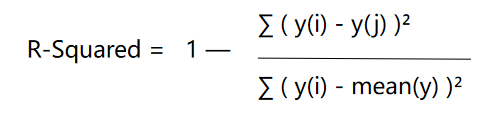def r2_score(y_test, y_predict):
""" R**2 """
assert len(y_test) == len(y_predict), "the length of y_test must be equal to y_predict"
assert y_test.ndim == 1 and y_predict.ndim == 1, "the ndim of y_test and y_predict must be 1"

return 1 - (np.sum((y_test - y_predict) ** 2)) / (np.sum((y_test - np.mean(y_test)) ** 2))


该方法对应于 sklearn 库中的 r2_score( y_test , y_predict )
from sklearn.metrics import r2_score

前三种评测方法的结果绝大多数情况下都是一个大于 1 的数值
对于同一种算法而言，即使使用不同类型的数据训练，对不同类型的数据进行预测，根据前三种评测结果，无法得出谁好谁坏的结论
例如使用多元线性回归算法，分别预测下个月的销售额和下次考试的语文成绩，评测结果无法看出是预测的销售额准确，还是预测的语文成绩准确
R-Squared 评测方法 完美解决了这个问题

R-Squared 将评测结果转换到 0~1 之间（也有可能会小于0）

R-squared 的最大值为 1 ，最小值可能会小于0

∑ ( y(i) - y(j) )²  表示我们的训练模型的预测值产生的错误大小

∑ ( y(i) - mean(y) )²  表示直接使用BaseModel模型预测数据产生的错误

当评测值小于 0 时，表示目前的训练模型效果还不如 BaseModel，此时数据之间很有可能不存在线性关系

BaseModel ： 使用均值作为训练模型，即无论预测时传入的数据是什么，都预测为训练数据集的均值


展开全文机器学习 python 数据挖掘
• 交互式简单线性回归 输入2D数据点并使用梯度下降拟合简单的线性回归模型。 使用构建。 可在播放。 文献资料 有关完整的文章，请访问。 开始使用 git clone git@github....
• 简单线性回归 机器学习 (MACHINE LEARNING) After finishing with the deep history and introduction to machine learning, I am hungry now and want to make some pancakes. But wait! every time I make ...
简单线性回归 机器学习 (MACHINE LEARNING)
After finishing with the deep history and introduction to machine learning, I am hungry now and want to make some pancakes. But wait! every time I make pancakes, I either overcook or undercook them. I wonder if machine learning can help me in making better pancakes by predicting the time for which I need to cook them? 在了解了很深的历史并介绍了机器学习之后，我现在很饿，想做点煎饼。 可是等等！ 每次我做薄煎饼时，我都会煮过或煮不到。 我想知道机器学习是否可以通过预测需要煮的时间来帮助我制作更好的煎饼？
From statistics, we know that correlation is a technique that can show how and how much pairs of variables are interrelated with each other. In simple words, how a change in one variable related to a component induces a change in the other variable of the same. 从统计数据中，我们知道关联是一种可以显示变量对如何相互关联的技术。 简而言之，与组件相关的一个变量的更改如何引起该组件的另一个变量的更改。
The proportion of ingredients, the thickness of pancakes, the temperature of the stove, the thickness of pan, humidity level, size of chimney, number of birds in the sky and many other factors are inducing a change in the cooking time of my pancakes. How to know which factor is most correlated? 食材的比例，煎饼的厚度，炉子的温度，锅的厚度，湿度，烟囱的大小，天空中的鸟类数量以及许多其他因素正在改变我的煎饼的烹饪时间。 如何知道哪个因素最相关？
There are different types of correlation techniques that help in determining the extent of known factors being interrelated with another factor. 有多种类型的相关技术可帮助确定已知因素与另一因素相关的程度。
Pearson r correlation: This is the most widely used correlation statistic to measure the degree of the relationship between linearly related variables. We can calculate r by using the below formula 皮尔逊相关性：这是使用最广泛的相关性统计量，用于度量线性相关变量之间的关系程度。 我们可以使用以下公式计算r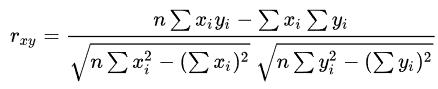rxy = Pearson r correlation coefficient between x and yn = number of observationsxi = value of x (for ith observation)yi = value of y (for ith observation) rxy =皮尔逊r x和y之间的相关系数n =观测次数xi = x的值(对于第i个观测值) yi = y的值(对于第i个观测值)
Kendall rank correlation: It is a non-parametric test that is used to measure the strength of interrelation between two variables. Considering two samples, a and b, where each sample size is n, we get the total number of pairings with a b equal to n(n-1)/2. Kendall rank correlation can be calculated using the below formula: 肯德尔等级相关：这是一种非参数检验，用于测量两个变量之间的相互关系强度。 考虑两个样本a和b，其中每个样本大小为n ，我们得到ab等于n ( n -1)/ 2的配对总数。 肯德尔等级相关性可以使用以下公式计算：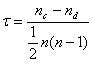nc= number of concordantnd= Number of discordant nc =一致数=不一致数 The above-explained techniques help us in determining the known variables which are influencing the unknown variable the most. In my pancake’s case, it is its thickness. Now, I just have to predict the time I need to cook them with respect to its thickness. 以上解释的技术有助于我们确定对未知变量影响最大的已知变量。 以我的煎饼为例，就是它的厚度。 现在，我只需要根据其厚度来预测烹饪时间。
Linear regression is a technique that helps us in modelling the relationship between the known variable (independent variable) and unknown variable (dependent variable) linearly. That is what will be the value of the dependent variable when the value of an independent variable is changing. 线性回归是一种帮助我们线性建模已知变量(独立变量)和未知变量(因变量)之间关系的技术。 当自变量的值改变时，这就是因变量的值。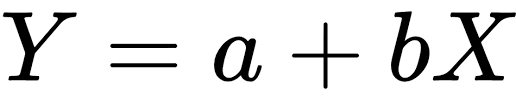Y = dependent variable Y =因变量
X = independent variable X =自变量
a = random error a =随机误差
b = regression coefficient b =回归系数
Now, that i know the independent variable which is inducing change to the dependent variable and the logic of Linear Regression, I can move ahead and build a model that can predict the time to cook a pancake. 现在，我知道了导致变量变化的自变量和线性回归的逻辑，我可以继续构建一个可以预测煮煎饼时间的模型。
Should I code it using Python or should I code it using R, I can use Java too. (The options while selecting a language for coding a machine learning model is overwhelming) 我应该使用Python编码还是使用R编码，我也可以使用Java。 (在选择用于编码机器学习模型的语言时，选项太多了)
Python is the most popular programming language used for coding, it has plenty of libraries like Teano, Keras, scikit-learn and TensorFlow making it easier for beginners of Machine Learning. Python是用于编码的最流行的编程语言，它具有诸如Teano，Keras，scikit-learn和TensorFlow之类的大量库，这使得机器学习的初学者更加容易。 R is mostly used for data analysis and statistics computation. It is best for data visualization and can also be used for Regression and Classification. R主要用于数据分析和统计计算。 它最适合用于数据可视化，也可以用于回归和分类。 Using Java provides simplification to large projects, better user interaction and is the most secure language due to its byte-code and sandboxes. 使用Java可以简化大型项目，实现更好的用户交互，并且由于其字节码和沙箱而成为最安全的语言。 I am using Python for making my Regression model predict the time for cooking my pancakes. You can choose any language based on the requirements. 我正在使用Python使我的回归模型预测烹饪薄煎饼的时间。 您可以根据要求选择任何语言。
First I need to import the data libraries 首先，我需要导入数据库 # importing data librariesimport numpy as npimport matplotlib.pyplot as pltimport pandas as pdNumpy — Numpy provides a high-performance multidimensional array and basic tools to compute with and manipulate the arrays Numpy — Numpy提供了高性能的多维数组和基本工具，可用于计算和操作数组
matplotlib.pyplot — matplotlib. pyplot is a collection of command style functions that make matplotlib work like MATLAB matplotlib.pyplot — matplotlib。 pyplot是使matplotlib像MATLAB一样工作的命令样式函数的集合
pandas — pandas offer data structures and operations for manipulating numerical tables and time series. 熊猫 -熊猫提供用于操纵数字表和时间序列的数据结构和操作。
2. Then, we need to import the dataset that we are using to train our regression model. I am importing a .csv file hence I am using .read_csv() function. 2.然后，我们需要导入用于训练回归模型的数据集。 我正在导入.csv文件，因此正在使用.read_csv()函数。
# importing datadataset = pd.read_csv(‘Pancakes_Data.csv’)X = dataset.iloc[:, :-1].valuesY = dataset.iloc[:,1].values3. Now, we need to split the imported dataset into training and test dataset, we usually prefer 2:1 ratio for the size of training and test dataset. 3.现在，我们需要将导入的数据集分为训练和测试数据集，对于训练和测试数据集的大小，我们通常首选2：1的比率。
# Splitting the dataset into training and test setsfrom sklearn.model_selection import train_test_splitX_tain, X_test, Y_train, Y_test = train_test_split(X, Y, test_size = 1/3, random_state = 0 )4. To make sure that the values in my data set are not too large or not too small, I will use the feature scaling technique. It is basically used for normalising the range of independent variables of data and is a general step of data preprocessing. 4.为确保数据集中的值不会太大或太小，我将使用要素缩放技术。 它基本上用于标准化数据自变量的范围，并且是数据预处理的一般步骤。
# Feature Scalingfrom sklearn.preprocessing import StandardScalersc_X = StandardScaler()X_tain = sc_X.fit_transform(X_tain)X_test = sc_X.transform(X_test)5. Now, this the step where our regression model trained, we are doing this by importing the LinearRegression from skLearn library. 5.现在，这是我们的回归模型训练的步骤，我们通过从skLearn库中导入LinearRegression来做到这一点。
# Fitting simple linear regression to the training setfrom sklearn.linear_model import LinearRegressionregressor = LinearRegression()regressor.fit(X_tain, Y_train)6. Since we are done with the training step, we can now predict the dependent variable using the trained regression model. 6.由于我们完成了训练步骤，因此我们现在可以使用训练后的回归模型预测因变量。
# Predicting the test set resultsY_pred = regressor.predict(X_test)BONUS STEP 奖励步骤
7. We can visualise the errors with the help of a scatter plot. 7.我们可以借助散点图可视化错误。
# Visualising the training set errorplt.scatter(X_tain, Y_train, color = ‘red’)plt.plot(X_tain, regressor.predict(X_tain), color = ‘blue’)plt.show()# Visualising the test set errorplt.scatter(X_test, Y_test, color = ‘red’)plt.plot(X_tain, regressor.predict(X_tain), color = ‘blue’)plt.show()Finally, I have a regression model that can predict the time needed to cook my pancakes. It will definitely help me in making better pancakes and it can help you too in solving linear real-world problems. 最后，我有一个回归模型，可以预测煮薄煎饼所需的时间。 它肯定会帮助我制作更好的煎饼，也可以帮助您解决线性现实问题。
We will continue to look at similar topics in future blogs as well. So stay tuned and let us know your thoughts on the article in the comment section below! 我们还将在以后的博客中继续关注类似的主题。 因此，请继续关注，并在下面的评论部分中告诉我们您对本文的看法！
翻译自: https://medium.com/image-vision/correlation-and-simple-linear-regression-9e9064c1fde0简单线性回归
展开全文python 机器学习 算法 人工智能 java
• 先考虑最简单的情形（简单线性回归）：输入属性的数目只有一个。简单线性回归线性回归试图学得 ，使得 我们可以通过使均方误差最小化来求w和b，即 均方误差对应了常用的欧氏距离。基于均方误差最小化来进...
• 简单线性回归中的加拿大房屋价格预测：使用BoxCox变换对要素进行简单线性回归中的加拿大房屋价格预测
• 线性回归是一种有监督机器学习算法，它分为简单线性回归和多元线性回归。从数学角度直观理解，它就是通过某个公式（比如y=a+bx1+cx2y=a+bx_1+cx_2y=a+bx1​+cx2​），来表达一个yyy值和nnn个xxx值之间的关系。 yyy值...机器学习 深度学习
• 梳理大纲： 简单线性回归和多元线性回归 【1】简单线性回归简单线性回归及最小二乘法的数据推导 【2】多元线性回归：多选线性回归和正规方程解及实现 参考资料: 机器学习 西瓜书 以下微信文章 From【木东居士】 ...
•机器学习...